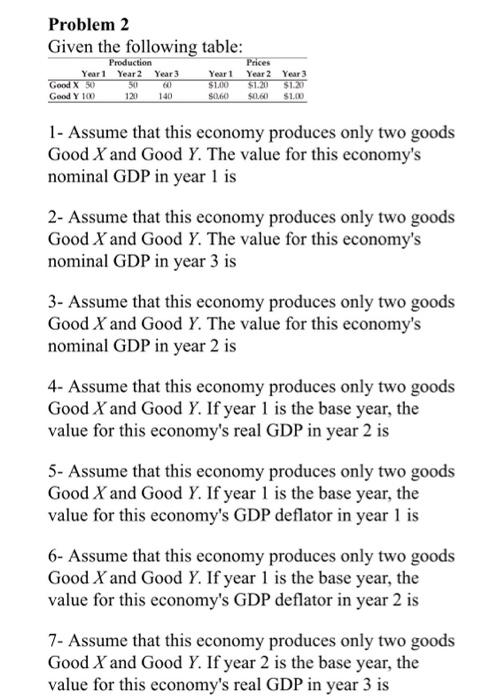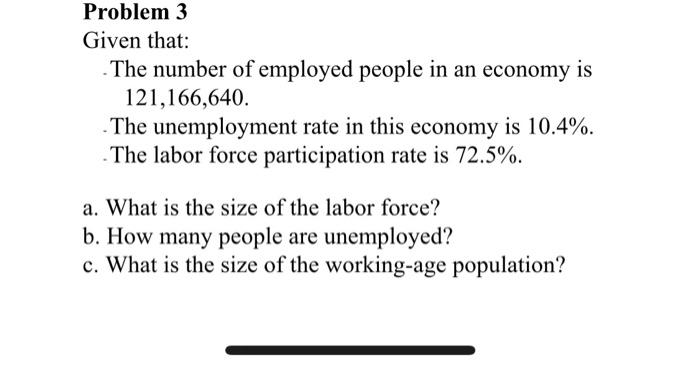### Create an Account

Already have account?

### Forgot Your Password ?

Home / Questions / Given the following table: Production Year 1 Year 2 Year 3 Good X 30 50 Good Y 100 Prices ...

# Given the following table: Production Year 1 Year 2 Year 3 Good X 30 50 Good Y 100 Prices Year 1 Year 2 Year 3 \$1200 \$1.20 \$1.27 50,60 \$0.60 \$1.00 123 1- Assume that this economy produces only two

Given the following table: Production Year 1 Year 2 Year 3 Good X 30 50 Good Y 100 Prices Year 1 Year 2 Year 3 \$1200 \$1.20 \$1.27 50,60 \$0.60 \$1.00 123 1- Assume that this economy produces only two goods Good X and Good Y. The value for this economy's nominal GDP in year 1 is 2- Assume that this economy produces only two goods Good X and Good Y. The value for this economy's nominal GDP in year 3 is 3- Assume that this economy produces only two goods Good X and Good Y. The value for this economy's nominal GDP in year 2 is 4- Assume that this economy produces only two goods Good X and Good Y. If year 1 is the base year, the value for this economy's real GDP in year 2 is 5- Assume that this economy produces only two goods Good X and Good Y. If year 1 is the base year, the value for this economy's GDP deflator in year 1 is 6- Assume that this economy produces only two goods Good X and Good Y. If year 1 is the base year, the value for this economy's GDP deflator in year 2 is 7- Assume that this economy produces only two goods Good X and Good Y. If year 2 is the base year, the value for this economy's real GDP in year 3 is
Problem 3 Given that: The number of employed people in an economy is 121,166,640. . The unemployment rate in this economy is 10.4%. The labor force participation rate is 72.5%. a.Jun 05 2021 View more View Less

#### Answer (Solved)Subscribe To Get Solution